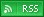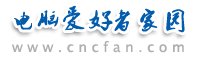电脑爱好者，提供IT资讯信息及各类编程知识文章介绍，欢迎大家来本站学习电脑知识。 最近更新 | 联系我们站内搜索：

# Asp之Base64加密解密代码

<%
OPTION EXPLICIT
const BASE_64_MAP_INIT = "ABCDEFGHIJKLMNOPQRSTUVWXYZabcdefghijklmnopqrstuvwxyz0123456789+/"
dim newline
dim Base64EncMap(63)
dim Base64DecMap(127)
'初始化函数
PUBLIC SUB initCodecs()
' 初始化变量
newline = "<P>" & chr(13) & chr(10)
dim max, idx
max = len(BASE_64_MAP_INIT)
for idx = 0 to max - 1
Base64EncMap(idx) = mid(BASE_64_MAP_INIT, idx + 1, 1)
next
for idx = 0 to max - 1
Base64DecMap(ASC(Base64EncMap(idx))) = idx
next
END SUB
'Base64加密函数
PUBLIC FUNCTION base64Encode(plain)
if len(plain) = 0 then
base64Encode = ""
exit function
end if
dim ret, ndx, by3, first, second, third
by3 = (len(plain) \ 3) * 3
ndx = 1
do while ndx <= by3
first  = asc(mid(plain, ndx+0, 1))
second = asc(mid(plain, ndx+1, 1))
third  = asc(mid(plain, ndx+2, 1))
ret = ret & Base64EncMap(  (first \ 4) AND 63 )
ret = ret & Base64EncMap( ((first * 16) AND 48) + ((second \ 16) AND 15 ) )
ret = ret & Base64EncMap( ((second * 4) AND 60) + ((third \ 64) AND 3 ) )
ret = ret & Base64EncMap( third AND 63)
ndx = ndx + 3
loop
if by3 < len(plain) then
first  = asc(mid(plain, ndx+0, 1))
ret = ret & Base64EncMap(  (first \ 4) AND 63 )
if (len(plain) MOD 3 ) = 2 then
second = asc(mid(plain, ndx+1, 1))
ret = ret & Base64EncMap( ((first * 16) AND 48) + ((second \ 16) AND 15 ) )
ret = ret & Base64EncMap( ((second * 4) AND 60) )
else
ret = ret & Base64EncMap( (first * 16) AND 48)
ret = ret '& "="
end if
ret = ret '& "="
end if
base64Encode = ret
END FUNCTION
'Base64解密函数
PUBLIC FUNCTION base64Decode(scrambled)
if len(scrambled) = 0 then
base64Decode = ""
exit function
end if
dim realLen
realLen = len(scrambled)
do while mid(scrambled, realLen, 1) = "="
realLen = realLen - 1
loop
dim ret, ndx, by4, first, second, third, fourth
ret = ""
by4 = (realLen \ 4) * 4
ndx = 1
do while ndx <= by4
first  = Base64DecMap(asc(mid(scrambled, ndx+0, 1)))
second = Base64DecMap(asc(mid(scrambled, ndx+1, 1)))
third  = Base64DecMap(asc(mid(scrambled, ndx+2, 1)))
fourth = Base64DecMap(asc(mid(scrambled, ndx+3, 1)))
ret = ret & chr( ((first * 4) AND 255) +   ((second \ 16) AND 3))
ret = ret & chr( ((second * 16) AND 255) + ((third \ 4) AND 15))
ret = ret & chr( ((third * 64) AND 255) +  (fourth AND 63))
ndx = ndx + 4
loop
if ndx < realLen then
first  = Base64DecMap(asc(mid(scrambled, ndx+0, 1)))
second = Base64DecMap(asc(mid(scrambled, ndx+1, 1)))
ret = ret & chr( ((first * 4) AND 255) +   ((second \ 16) AND 3))
if realLen MOD 4 = 3 then
third = Base64DecMap(asc(mid(scrambled,ndx+2,1)))
ret = ret & chr( ((second * 16) AND 255) + ((third \ 4) AND 15))
end if
end if
base64Decode = ret
END FUNCTION
' 初始化
call initCodecs
' 测试代码
dim inp, encode
inp = "1234567890"
encode = base64Encode(inp)
response.write "加密前为:" & inp & newline
response.write "加密后为:" & encode & newline
response.write "解密后为:" & base64Decode(encode) & newline
%>
 Asp热门文章排行 网站赞助商